# Functions for lookup of data

These functions perform a lookup on a data range using the provided value and return the result.

Returns the cell reference for the given row and column.

Example:

#### CELLS

Returns the number of cells in the given cell range or data array.

CELLS(data)

Example:
CELLS(A1:C4) = 12

#### CELLVALUE

Returns the value in the cell with the given cell reference. This is only relevant in models and is useful in cell formulas and for referencing data in import queries.

CELLVALUE(cell)

Example:
CELLVALUE("C2") = 25

#### CHOOSE

Returns the value of the given index in the given cell range, data array, or comma-delimited list of values.

CHOOSE(data, index)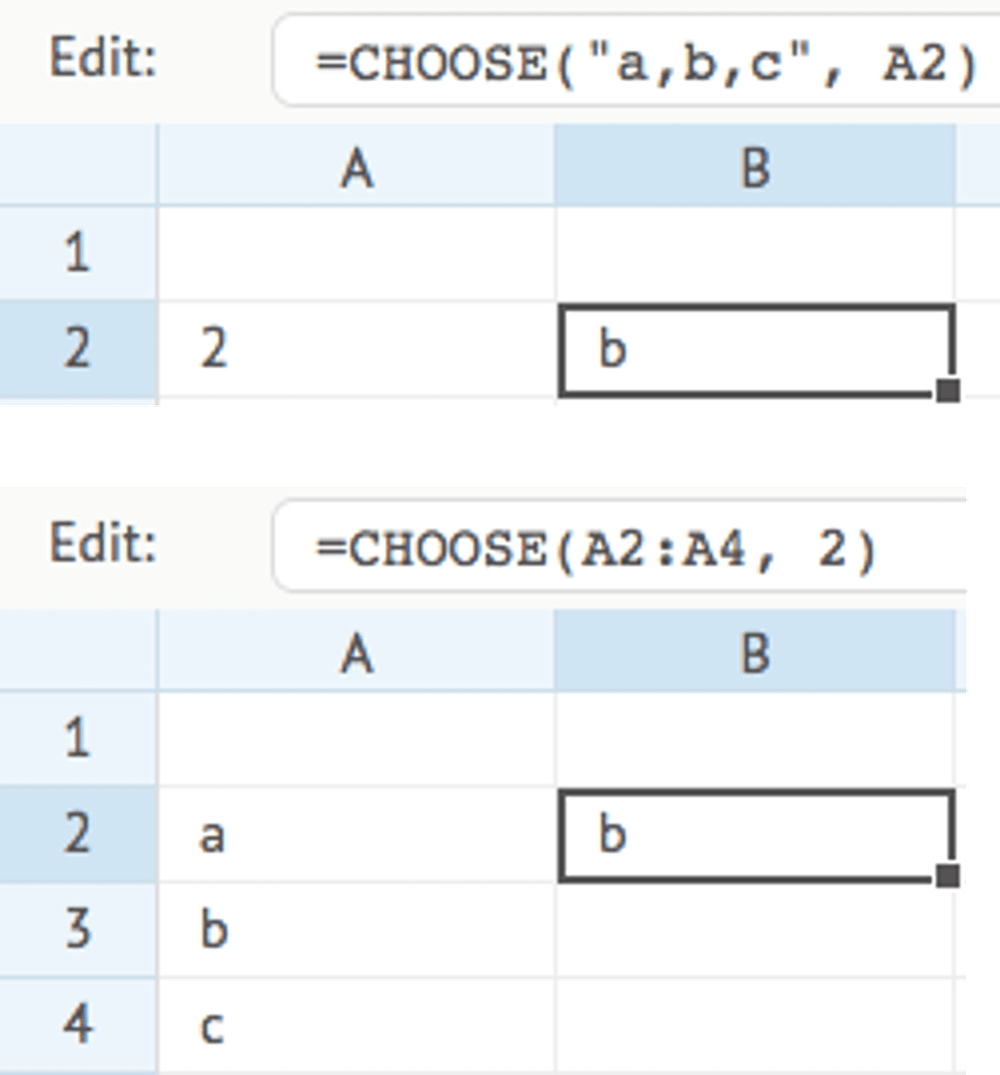#### COLUMNS

Returns the number of columns in the given cell range or data array. The range must contain only one row.

COLUMNS(data)

Example:
COLUMNS(A1:E1) = 5

#### COMPARE

Compares the values in the 2 given cell ranges or data arrays, and returns the outcome defined by the "type" parameter. Both ranges need to be of comparable size. The common use-case for this function is to understand the difference in values between 2 ranges.

COMPARE(data, range, type)

Type:
0 - returns true if the values in both ranges are the same, else returns false (default)
1 - returns the number of values that differ between both ranges
2 - returns the changed values as a comma-delimited list
3 - returns the changed values and the compared values as a comma-delimited list

Example:
COMPARE(A1:A5, B1:B5, 3) = "Peter Smith (John Smith)"

#### DISTINCT

Returns a list with the sorted unique values that occur in the given cell range, data array, or comma-delimited list of values.

DISTINCT(data)

Example:
data = "a,a,b,a,c,b,c,b,a"
DISTINCT(data) = "a,b,c"

#### EXTRACT

Returns the value or text that resides between the 2 given keys. This function is useful to extract words from texts, or to extract values from within HTML or XML tags.

EXTRACT(data, key, endkey)

Example:
data = "<product>coffee</product> "
EXTRACT(data, "<product>", "</product>") = "coffee"

#### FIND

Returns the starting position of a given text string (the "key" parameter) within another text string (the "data" value).

FIND(data, key)

Example:
data = "this text"
FIND(data, "tex") = 6

#### FIRSTVALUE

Returns the first non-blank value in the given cell range of data array.

FIRSTVALUE(data)

Example:
FIRSTVALUE("", "a", "", "b", "") = "a"

#### HLOOKUP

Looks up the given "key" value in the given horizontal cell range or data array ("data"), and returns the value in the same column in the second horizontal cell range or data array ("range").

The lookup will always try an exact match on the "key" value, and return an empty text when no exact match is found. If you want to find the nearest or approximate match when no exact match is found, you need to set the "type" parameter.

HLOOKUP(data, range, key, type)

Type:
0 - exact match only (default)
1 - find the next value just after the given key
2 - find the previous value just before the given key
3 - case-insensitive fuzzy match by ignoring all characters other than A-Z and 0-9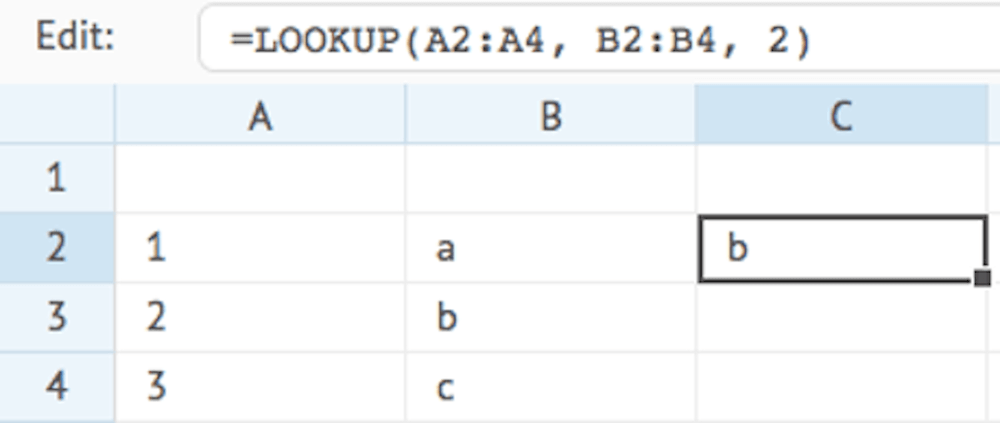#### INDEX

Returns a single value from the given cell range or data array, based on the given index. The index is a sequential result of the combined row and column position. For example, a range with 3 rows and 2 columns has 6 indices (3 * 2). The value on row 2 and column 1 has index 3 (1 * 2 + 1), whilst the value on row 3 and column 2 has index 6 (2 * 2 + 2), and so on.

INDEX(data, index)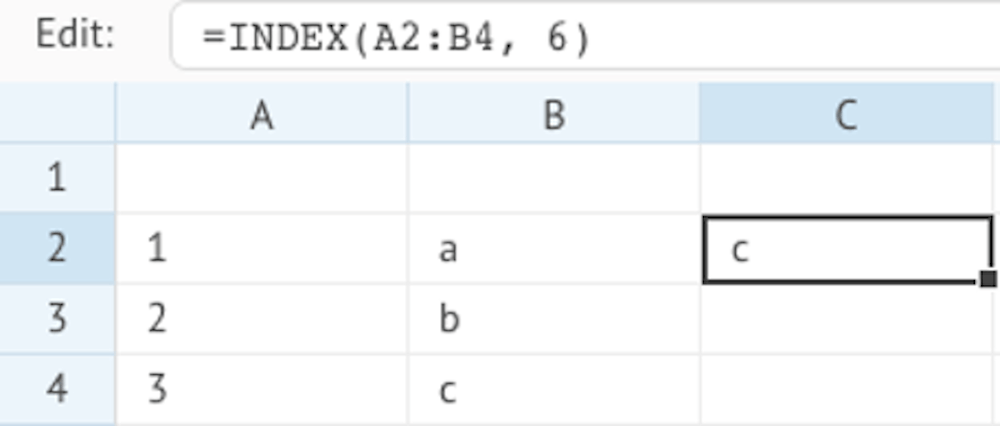#### INDIRECT

Returns the value in the cell with the given cell reference, or with the given row and column. This is only relevant in models and enables you to indirectly obtain the value in a cell using a dynamic reference. This enables you to dynamically change the reference to a cell within a formula without having the change the formula itself.

INDIRECT(cell) or INDIRECT(row, column)

Example:
INDIRECT("C2") = 25
INDIRECT(2, 3) = 25

#### JSON

Returns a specific property value from a JSON-formatted text. You can specify the nodes(s) that represent the values you want. You can specify nested nodes by a period, e.g. mydata.product.description. You can also specify array positions, e.g. mydata.products.0.id

JSON(data, key)

Example:
data = {"mydata":{"product":{"id":"1","description":"coffee","price":"10"}}}
JSON(data, "mydata.product.description") = "coffee"

#### LABEL

Returns a text label for the given index by looking it up in the given cell range, data array, or comma-delimited list of values.

LABEL(data, index)

Example:
LABEL("Jan,Feb,Mar", 2) = "Feb"

#### LASTVALUE

Returns the last non-blank value in the given cell range of data array.

LASTVALUE(data)

Example:
LASTVALUE("", "a", "", "b", "") = "b"

#### LOOKUP

Looks up the given "key" value in the given cell range or data array ("data"), and returns the value on the same row in the second cell range or data array ("range").

The lookup will always try an exact match on the "key" value, and return an empty text when no exact match is found. If you want to find the nearest or approximate match when no exact match is found, you need to set the "type" parameter.

LOOKUP(data, range, key, type)

Type:
0 - exact match only (default)
1 - find the next value just after the given key
2 - find the previous value just before the given key
3 - case-insensitive fuzzy match by ignoring all characters other than A-Z and 0-9#### MATCH

Compares each item in the given cell range or data array ("data") to a condition, and returns the value on the first row in the second cell range or data array ("range") that matches the condition.

MATCH(data, range, condition)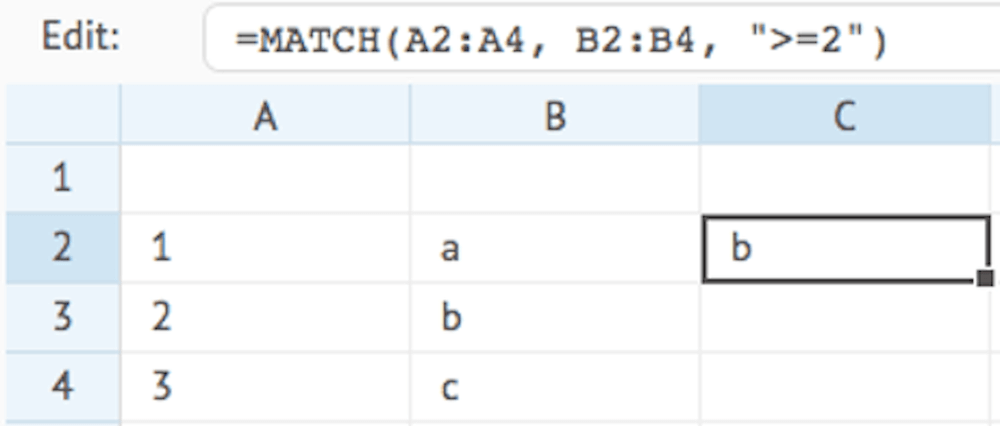#### PLANNER

Returns a proportion of data according to the selected planner and the selected index. The returned value is calculated as follows:

data * value of given planner index / sum of all planner values

If the given index does not exist in the planner, the function will use the given ratio (which can be a cell reference, a formula, or a given value) and will return the result of data * ratio.

If neither the index or a ratio exists, the function will return 0 (zero).

PLANNER(data, planner, index, ratio)

Examples:
PLANNER(1000, "Months", 6) = 83.33  (1000 * 1 / 12
PLANNER(1000, "Weeks445", 6)    = 96.15  (1000 * 5 / 52)
PLANNER(1000, "Summmer", 6)     = 111.11 (1000 * 4 / 36)
PLANNER(1000, "", 0, B3/C3)     = 200.00 (1000 * 1 / 10

The PLANNER function works in conjunction with the Cell editor Planner and enables you to distribute a given value across a range of cells in a model, based on a defined pattern that can be selected by the user. For example, this can be used to divide an given amount over a range of periods, or across products etc.

When you want to use a PLANNER function, you first need to define a Cell editor Planner.

The below example defines 3 patterns for a cell editor: 6 equal months, a Summer pattern, and a Winter pattern: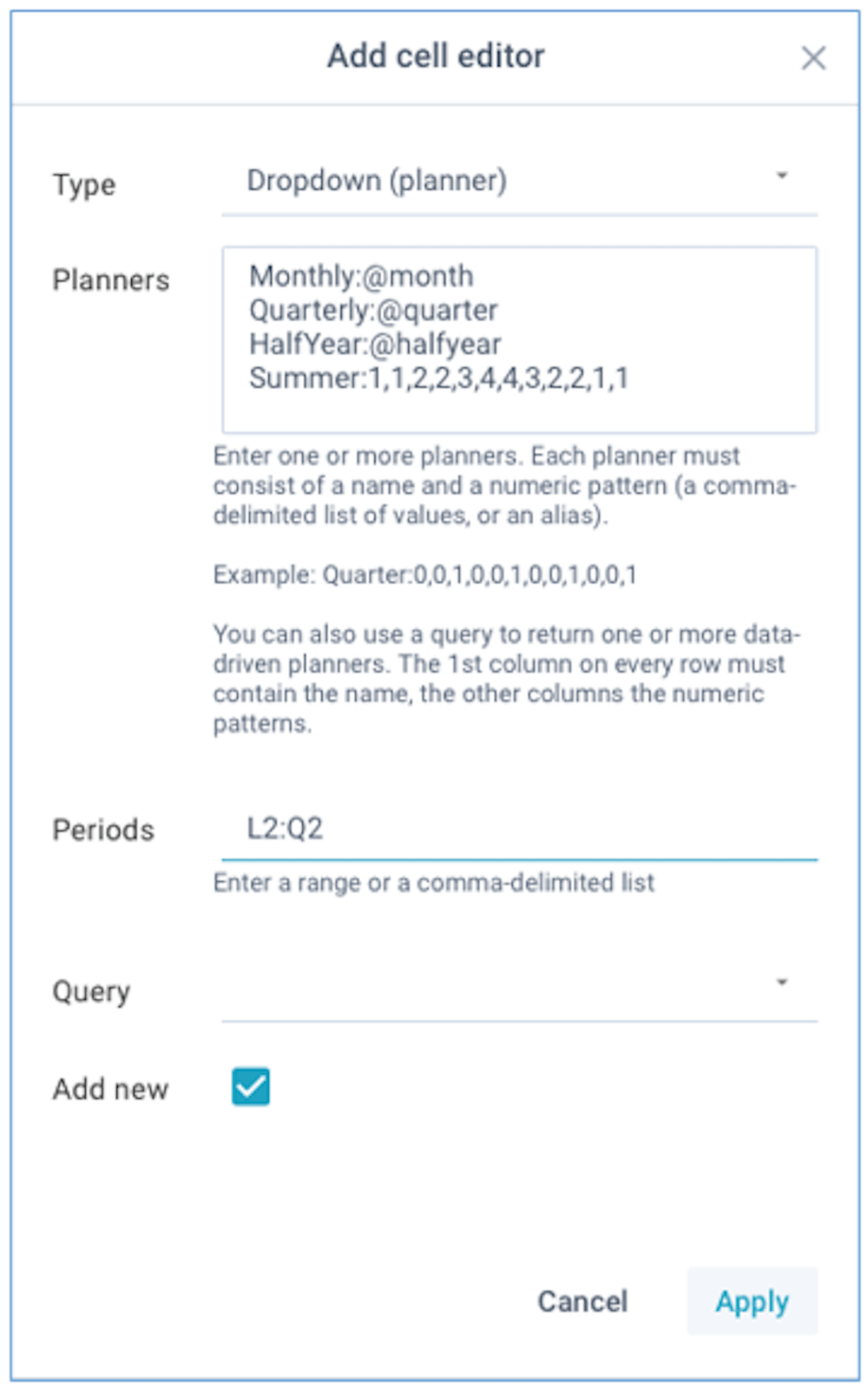The absolute values of the numeric patterns are irrelevant, what matters is each relative value as a proportion of the sum of all values. You can define as many indices in the patterns as you need.

You can also use one of the below aliases for common patterns:

• @month - every month (1,1,1,1,1,1,1,1,1,1,1,1)
• @quarter - every quarter (0,0,1,0,0,1,0,0,1,0,0,1)
• @soquarter - start of quarter (1,0,0,1,0,0,1,0,0,1,0,0)
• @halfyear - every half year (0,0,0,0,0,1,0,0,0,0,0,1)
• @sohalfyear - start of half year (1,0,0,0,0,0,1,0,0,0,0,0)
• @year - every year (0,0,0,0,0,0,0,0,0,0,0,1)
• @soyear - start of year (1,0,0,0,0,1,0,0,0,0,0,0)
• @period445 - 445 week-based (4,4,5,4,4,5,4,4,5,4,4,5)

In addition to defined static planners, you can also refer to a data query. Your data query can include multiple rows, with each row being treated as a separate planner. The first column on every row needs to contain the planner name, and the other columns need to contain the numeric patterns.

#### RANK

Returns the rank (starting at 1) of a given number within the list of numbers in the given cell range or data array. You can decide if/how the list will be sorted.

RANK(data, number, type)

Type:
"asc"  - ascending sort (default)
"desc" - descending sort
"none" - no sort

Example:
RANK(A1:A4, 45, "asc") = 3

#### ROWS

Returns the number of rows in the given cell range or data array. The range must contain only one column.

ROWS(data)

Example:
ROWS(A1:A4) = 4

#### SWITCH

Returns the corresponding value that matches the given key in the given cell range or data array, or comma-delimited list of values. The list must contain the keys and their corresponding values as separate consequtive items. If no value is found, either the key is returned, or an empty value if you set that parameter to true.

SWITCH(data, key, empty)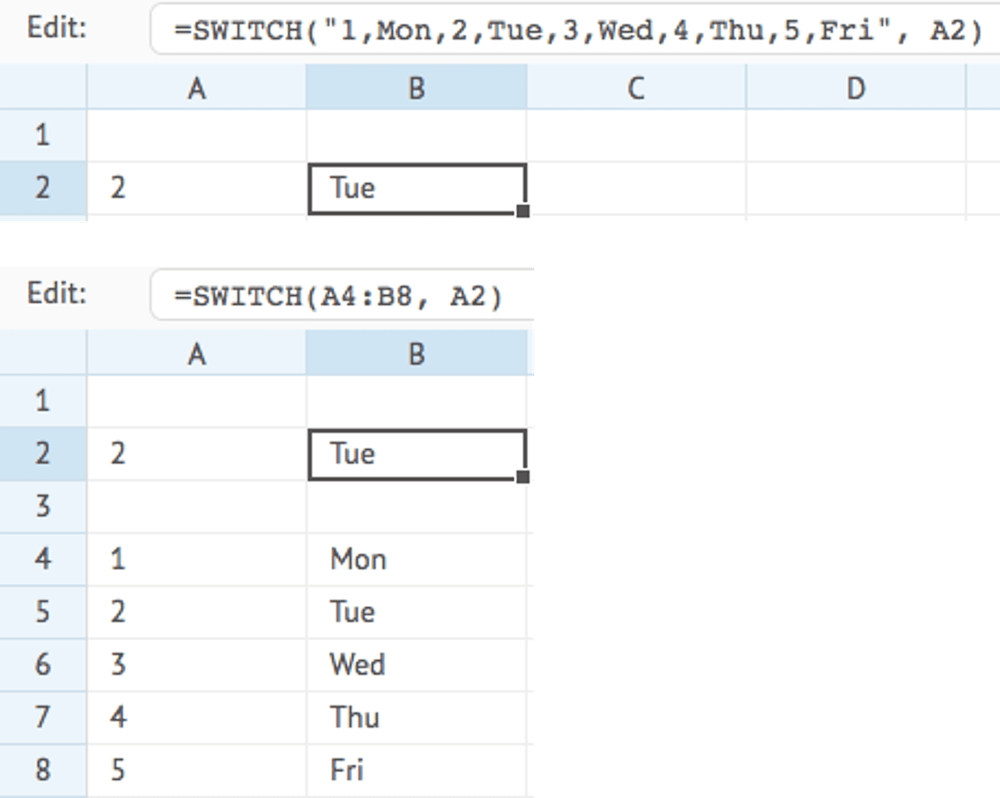#### VLOOKUP

Looks up the given "key" value in the given vertical cell range or data array, and returns the value on the same row in the second horizontal cell range or data array ("range").

The lookup will always try an exact match on the "key" value, and return an empty text when no exact match is found. If you want to find the nearest or approximate match when no exact match is found, you need to set the "type" parameter.

VLOOKUP(data, range, key, type)

Type:
0 - exact match only (default)
1 - find the next value just after the given key
2 - find the previous value just before the given key
3 - case-insensitive fuzzy match by ignoring all characters other than A-Z and 0-9#### WEBSERVICE

Returns data from a web service on the Internet or Intranet. Only connections over https:// are allowed.

If the data is returned in JSON format, you can specify the JSON node(s) that contains the required value(s). You can specify nested nodes by a period, e.g. mydata.product.description. You can also specify array positions, e.g. mydata.products..id

If you don't specify any node, the entire JSON data will be returned as text and you can use the JSON function to extract the necessary value(s) from that data.

If the data is returned in text, XML, or HTML format, you can use standard lookup and text functions (e.g. FIND, EXTRACT, LEFT, MID, RIGHT etc) to extract the required value(s).

WEBSERVICE(url, key)

Example:
WEBSERVICE("https://api.exchangeratesapi.io/latest", "rates.USD")

#### WEBVIEW

Views the content of a web page on the Internet or Intranet. You can optionally define the width and height of the window that contains the content.

WEBVIEW(url, width, height)

Example:
WEBVIEW("https://www.xlreporting.com")

#### WRAP

Wraps a given cell range or data array into a single JSON-formatted text. Ideal for storing complex data into a single value, for example when saving into a data set column. Each value is given a sequential numeric key starting at 1 (e.g. 1, 2, 3 etc) in order of the values passed. Use UNWRAP to extract the data again.

WRAP(data)

Example:
WRAP(A3:B3)
WRAP(A3, B3, C3)

#### UNWRAP

Returns a specific value from a single JSON-formatted text that was created by WRAP. The key is numeric in order of the values passed to WRAP (e.g. 1, 2, 3 etc). Use WRAP to create the JSON-formatted text.

UNWRAP(data)

Example:
UNWRAP(data, 2)